# C语言详解：指针

### 指针

#### 指针定义

##### 内存划分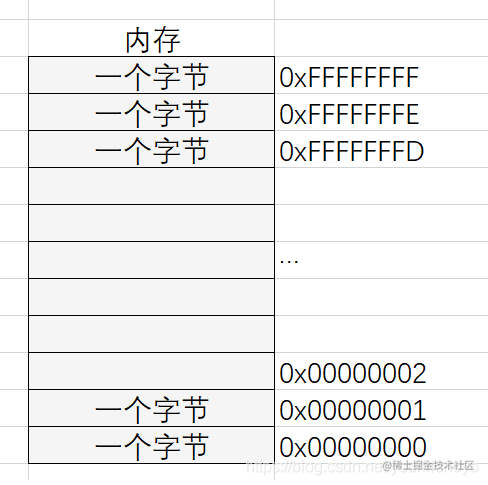##### 指针与指针变量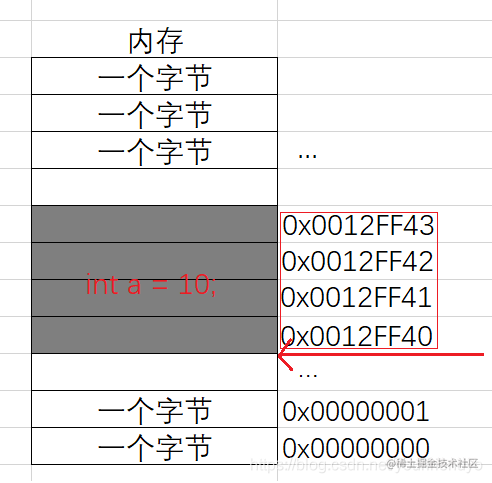int * pa = &a;

##### 总结
1. 指针即地址，地址即指针。
2. 指针变量是存放地址的变量，其中的内容都被当作地址处理。

##### 指针大小
• 一个内存单元有多大？
• 地址是如何进行编号？

00000000 00000000 00000000 00000000

00000000 00000000 00000000 00000001

… …

11111111 11111111 11111111 11111110

11111111 11111111 11111111 11111111

#### 指针类型

int a = 10;
int * pa = &a;

• * 代表 pa 是指针
• int 代表pa所指向的变量类型为int

##### 指针解引用方面
int a = 0x11223344;
int* pa = &a;
*pa = 0;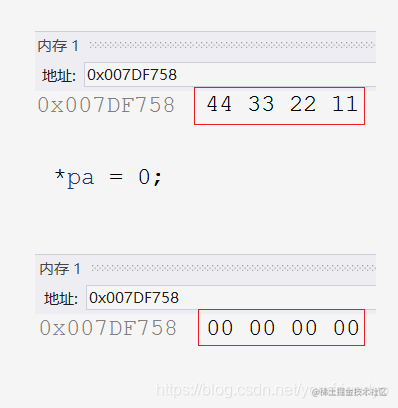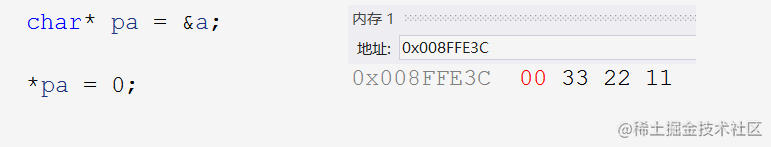##### 指针±正数方面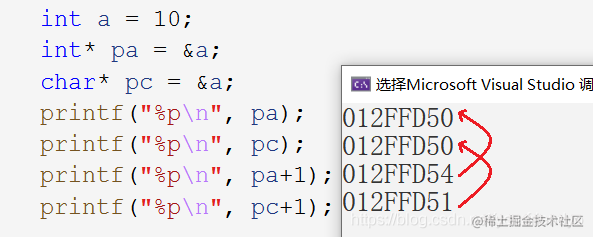##### 总结

1. 指针解引用操作时能够访问的字节（内存大小）。
2. 指针±整数时能跳过几个字节（步长）。

int arr = { 0 };
//1.
int* pa = arr;
//2.
char * pa = arr;
for (int i = 0; i < 10; i++)
{
*(pa + i) = 1;
}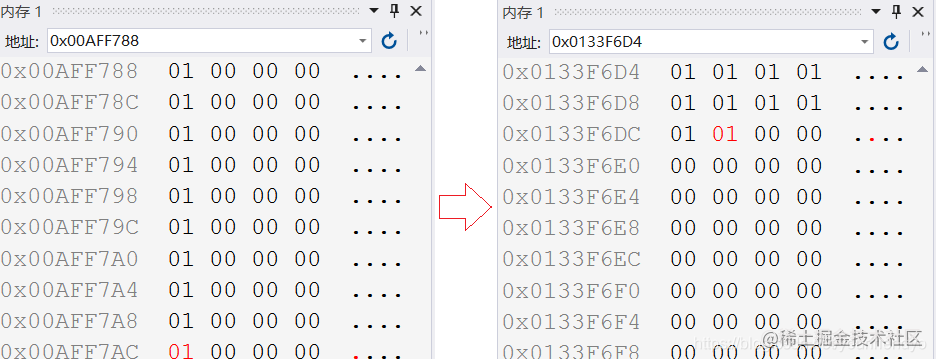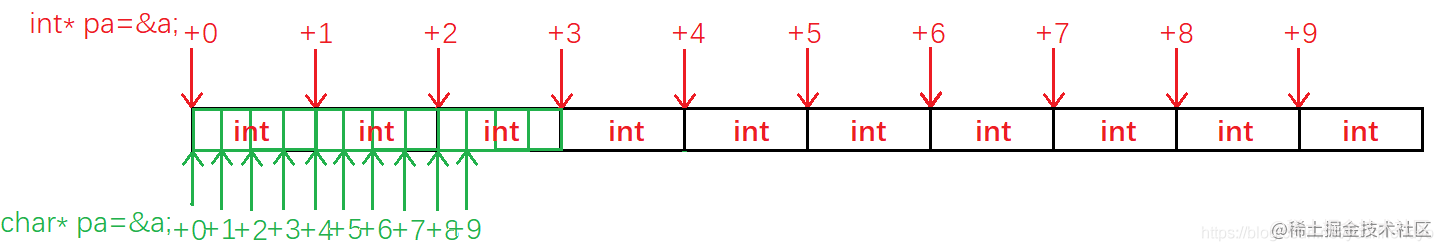#### 野指针

##### 野指针成因
1. 指针未初始化

 int* p;//未初始化
*p = 20;
复制代码
2. 指针越界访问

int arr = { 0 };
int* p = arr;
for (int i = 0; i <= 10; i++)//越界访问
{
*(p + i) = i;
}
复制代码

• 例题

 int* test(){
int a = 10;
return &a;
}
int main(){
int* p = test();
printf("%d\n", *p);//野指针越界访问
return 0;
}
复制代码

• 我们稍作修改，在打印*p的前面再调用一次printf函数，如：
int* test(){
int a = 10;
return &a;
}
int main(){
int* p = test();
printf("hehe\n");
printf("%d\n", *p);
return 0;
}
复制代码

• 那如果我们把打印printf("%d\n", *p);改为赋值语句*p = 20;的话，如：

int* test(){
int a = 10;
return &a;
}
int main(){
int* p = test();
*p = 20;//访问非法内存
return 0;
}
复制代码

1. 指针指向空间已释放

##### 如何规避野指针
1. 明确指针初始化，确定指向

 int* p = &a;
int* p =NULL;//不知道该指向何处时，置为空NULL
复制代码
2. 谨防指针越界

3. 指针指向空间释后，立即置为NULL

4. 避免函数返回局部变量地址

5. 检查指针有效性

if(p != NULL){
*p=20;//检验不为空指针，再使用
}

#### 指针运算

##### 指针+-整数
float values[N_VALUE];
float* vp = values;
for (vp = &values; vp < &values[N_VALUE];)
{
*vp++=0;
}

• 循环体内，vp++*，尽管++的优先级比*要高，但是后置++是先使用再++。

所以仿佛是对指针先解引用再++的。

• float类型指针的加一，跳过一个float类型的长度，故跳到下一个元素。

不论指针是什么类型，指针++，都是跳过一个类型的长度。

• vp指向数组最后一个元素其后的地址，不满足条件，结束循环。

该地址虽不属于数组，但仅是用所判断大小的条件(地址有高低)，没有访问该地址的内容，所以不算越界访问。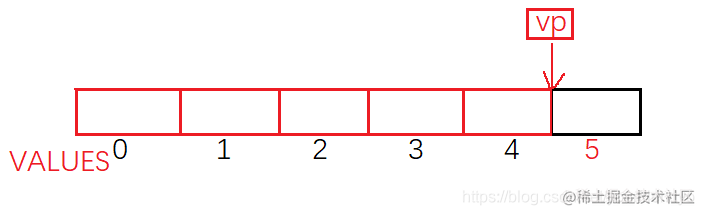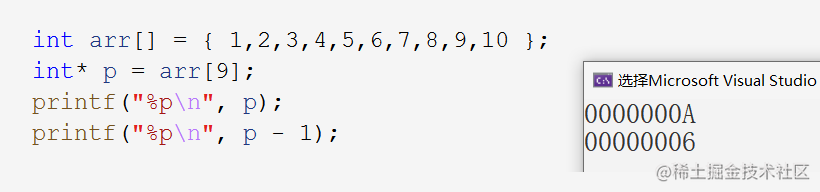##### 指针-指针

int arr[] = { 1,2,3,4,5,6,7,8,9,10 };
printf("%d\n", &arr - &arr);

int arr[] = { 1,2,3,4,5,6,7,8,9,10 };
char ch[] = { '1','2','3' };
printf("%d\n", &arr - &ch);

• 指针-指针的前提：是两指针指向同一块空间。

• 指针-指针，得到的数字的绝对值是两地址之间的元素个数。

int my_strlen(const char* s){
char* begin = s;//标记开头
while(*s++);//s先++再判断是否为\0
return s - begin - 1;//指针相减
}

##### 指针关系运算

//1.
for(vp = &values[N_VALUE];vp > &values;){
*--vp = 0;
}

//2.
for(vp = &values[N_VALUE-1];vp >= &values;vp--){
*vp = 0;
}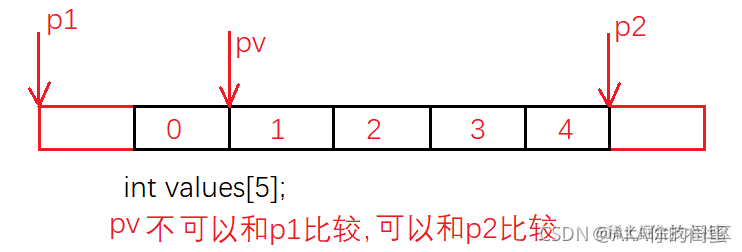#### 指针和数组

• 数组是一个相同类型元素的集合，其中元素存放在连续的空间中。数组的大小取决于元素类型和元素个数。

• 指针存储地址，是一个变量。指针的大小固定为4 (32bit) / 8 (64bit)。

int arr = { 0 };
printf("%p\n", arr);//0x0012ff40
printf("%p\n", &arr);//0x0012ff40

ps：以下两种情况数组名代表整个数组，除该两种情况外，数组名都代表首元素地址。

1. sizeof(arr)
2. &arr

int arr = { 1,2,3,4,5,6,7,8,9,10 };
int* p = arr;
for (int i = 0; i < sz; i++)
{
printf("&arr[%d] = %p <===> p+%d = %p\n", i, &arr[i], i, p + i);
}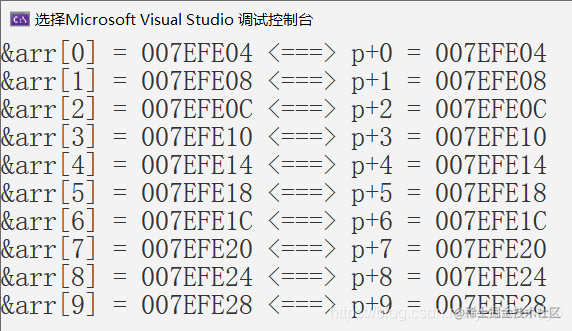#### 二级指针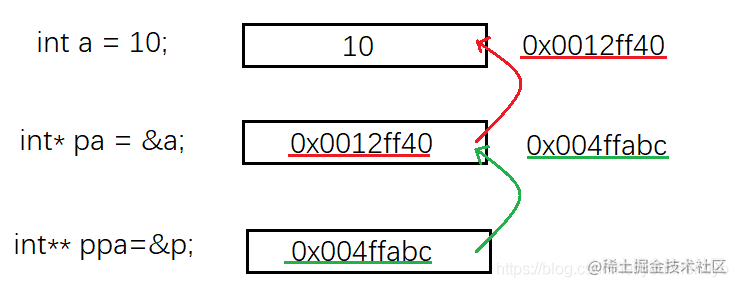1. 首先创建了一个变量a，存了10，所以它的类型为int ，变量的地址为0x0012ff40

2. 然后取出a的地址，再创建了一个新的变量pa，并把&a存了进去，所以它的类型为int*（一级指针），变量的地址为0x004ffabc

3. 最后又创建了一个新的变量ppa，把&p存了进去，所以它的类型为int**（二级指针）。

##### 类型中“*”的含义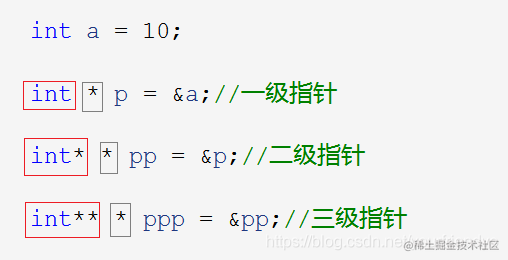• 一级指针p前面的int表示p指向的对象aint型的。
• 二级指针pp前面的int*表示pp指向的对象p的类型是int*型的。
• 三级指针ppp前面的int**表示ppp指向的对象pp的类型是int**型的。
##### 多级指针解引用操作
*p = 1;
* *pp = 2;
* * *ppp = 3;

• 对一级指针p解引用*p，找到a
• 对二级指针pp解引用*pp，找到p，再解引用**pp，找到a
• 对三级指针ppp解引用*ppp找到pp，再解引用**ppp，找到p，再解一次引用***ppp，找到a

#### 指针数组

##### 指针数组定义

int arr = {0};
//整型数组 - 存放整型变量的数组
char ch = {'0'};
//字符数组 - 存放字符变量的数组

//整型指针数组
int* parr;
//字符型指针数组
char* pch;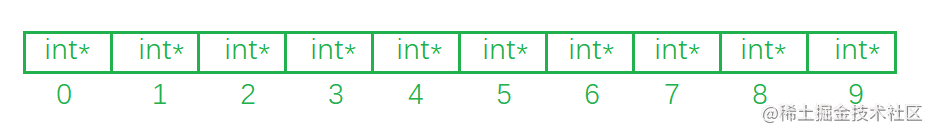##### 指针数组使用
int arr[] = { 10,20,30 };
int* parr = {NULL};
//输入
for (int i = 0; i < 3; i++)
{
parr[i] = &arr[i];
}
//输出1.
for (int i = 0; i < 3 ; i++)
{
printf("%d ", *parr[i]);
}
//输出2.
for (int i  = 0; i < 3; i++)
{
printf("%d ", **(parr+i));
}

1. 切记要么初始化要么指定大小。指针数组记得内容初始化为空指针。
2. 指针数组遍历数组元素打印时，记得要解引用。用数组名+i遍历数组元素时，就要解两层引用。